doclist 阅读(38) 评论(0)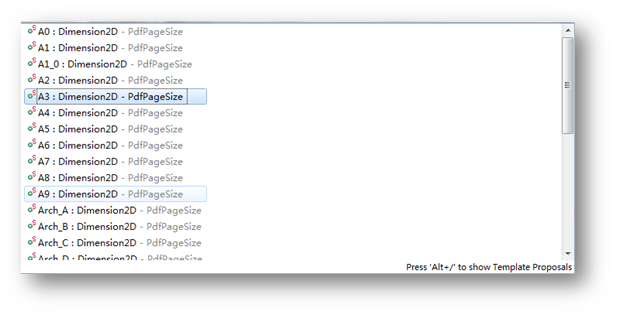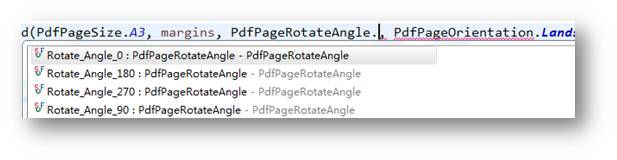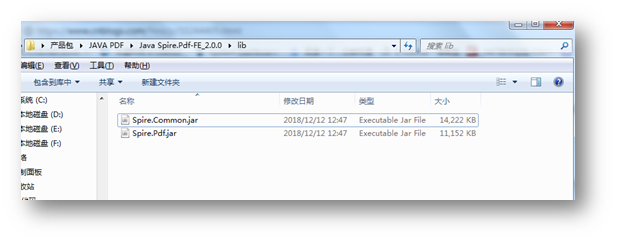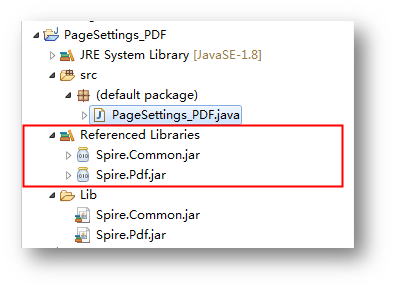Java示例（供参考）

```import com.spire.pdf.*;
import com.spire.pdf.graphics.PdfMargins;
import java.awt.*;
import java.awt.geom.Dimension2D;
import java.awt.geom.Point2D;

public class PageSettings_PDF {
public static void main(String[] args){
//创建PdfDocument对象
PdfDocument originalDoc = new PdfDocument();
//加载PDF文件
originalDoc.loadFromFile("input.pdf");

//创建一个新的PdfDocument实例
PdfDocument newDoc = new PdfDocument();

//遍历所有PDF 页面
Dimension2D dimension2D = new Dimension();
for (int i = 0; i < originalDoc.getPages().getCount(); i++) {
PdfPageBase page = originalDoc.getPages().get(i);
if (i == 0) {
//设置新文档第一页的页面宽、高为原来的1.2倍
float scale = 1.2f;
float width = (float) page.getSize().getWidth() * scale;
float height = (float) page.getSize().getHeight() * scale;
dimension2D.setSize(width, height);
//设置新文档第一页的页边距为左右50，上下100
PdfMargins margins = new PdfMargins(50, 100);
PdfPageBase newPage = newDoc.getPages().add(dimension2D, margins);
//复制原文档的内容到新文档
newPage.getCanvas().drawTemplate(page.createTemplate(), new Point2D.Float());
}

if (i == 1) {
//设置新文档第二页的页边距为左右100、上下100
PdfMargins margins = new PdfMargins(100,100);
//设置新文档第二页的页面大小为A3
PdfPageBase newPage = newDoc.getPages().add(PdfPageSize.A3, margins);
//调整画布，设置内容也根据页面的大小进行缩放
double wScale = (PdfPageSize.A3.getWidth() - 10) / PdfPageSize.A3.getWidth();
double hScale = (PdfPageSize.A3.getHeight() - 10) / PdfPageSize.A3.getHeight();
newPage.getCanvas().translateTransform(wScale, hScale);
//复制原文档的内容到新文档
newPage.getCanvas().drawTemplate(page.createTemplate(), new Point2D.Float());
}

if (i == 2) {
//设置新文档第三页的页边距为左右200，上下50
PdfMargins margins = new PdfMargins(240, 50);
//设置新文档第三页的页面大小为A3, 页面旋转角度为0，纸张方向为水平
PdfPageBase newPage = newDoc.getPages().add(PdfPageSize.A3, margins, PdfPageRotateAngle.Rotate_Angle_0, PdfPageOrientation.Landscape);
//调整画布，设置内容也根据页面的大小进行缩放
double wScale = PdfPageSize.A4.getHeight() / page.getSize().getWidth();
double hScale = PdfPageSize.A4.getWidth() / page.getSize().getHeight();
newPage.getCanvas().translateTransform(wScale, hScale);
//复制原文档的内容到新文档
newPage.getCanvas().drawTemplate(page.createTemplate(), new Point2D.Float());
}

//保存PDF
newDoc.saveToFile("pdfPageSetting.pdf");
}
}
}```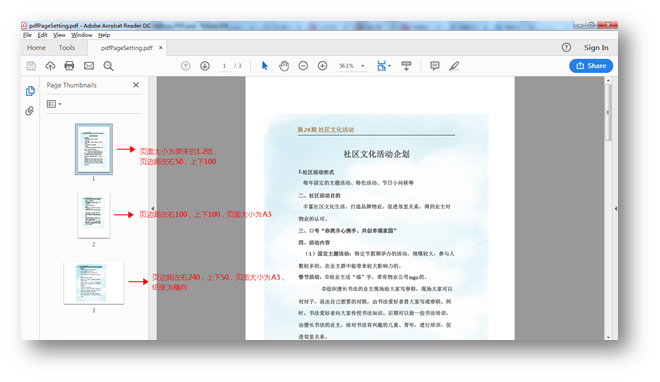（本文完）

转载请注明出处！